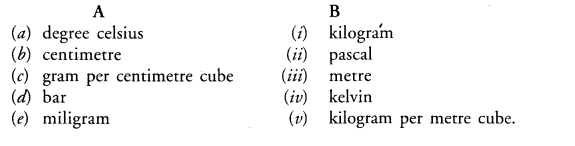# The non SI and Sl units of some physical`
Question:

The non $\mathrm{SI}$ and $\mathrm{Sl}$ units of some physical quantities are given in column $\mathrm{A}$ and column $\mathrm{B}$ respectively. Match the units belonging to the same physical quantity:Solution:

(a)—(iv),

(b)—(iii),

(c)—(v),

(d)—(ii),

(e)—(i)